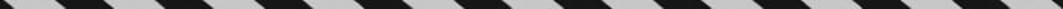﻿ ﻿ARITHMETIC OPERATIONS WITH MIXED NUMBERS - Fractions, Decimals, and Percents - TOPICS IN ARITHMETIC - SAT SUBJECT TEST MATH LEVEL 1

## TOPICS IN ARITHMETIC## CHAPTER 3Fractions, Decimals, and Percents### ARITHMETIC OPERATIONS WITH MIXED NUMBERS

A mixed number is a number such asthat consists of an integer followed by a fraction. It is an abbreviation for the sum of the integer and the fraction; so,.

Tactic B3

 TACTICB3 To do arithmetic with mixed numbers, just enter them into your calculator as sums written in parentheses.

EXAMPLE 9: How you evaluatedepends on your calculator. If you use a scientific calculator with a fraction button, you can enter. If you use a graphing calculator, you would probably enter it as (1 + 23)(3 + 14). Either way, you should getoror, which you can convert to the fraction.

﻿